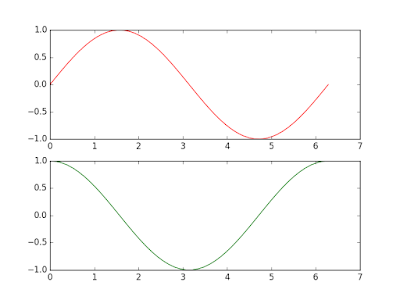Topics: AI | Data Science | Data Visualization | Deep Learning | Machine Learning | NLP | Python | R | Statistics

KDnuggets Home » News » 2016 » Jun » Tutorials, Overviews » An Introduction to Scientific Python (and a Bit of the Maths Behind It) – Matplotlib ( 16:n21 )

# An Introduction to Scientific Python (and a Bit of the Maths Behind It) – Matplotlib

An introductory overview of Matplotlib, one of the foundational aspects of Scientific Computing in Python, along with some explanation of the maths involved.

### Using Subplots

Subplots allow you to plot multiple graphs in one window.

```# Using subplot.
x = np.linspace(0, 2 * np.pi, 50)
plt.subplot(2, 1, 1) # (row, column, active area)
plt.plot(x, np.sin(x), 'r')
plt.subplot(2, 1, 2)
plt.plot(x, np.cos(x), 'g')
plt.show()
```

When using subplots, we plot datasets as in the previous examples but with one extra step. Before calling the plot() function, we first call the subplot() function. The first argument is the number of rows you want the subplot to have, the second is the number of columns and the third is the active area.The active area is the current subplot you are working on now and are numbered from left to right, up to down. For example in a 4x4 grid of subplots, the active area 6 would be (2,2) on the grid.You should have two graphs like this:### Simple Scatter Graphs

Scatter graphs are a collection of points that are not connected by a line. Again, Matplotlib makes this a trivial task.

```# Simple scatter plotting.
x = np.linspace(0, 2 * np.pi, 50)
y = np.sin(x)
plt.scatter(x,y)
plt.show()
```

As the above code shows, all you do is call the scatter() function and pass it two arrays of x and y coordinates. Note that this can also be reproduced by using the plot command with the line styling 'bo'.You should end up with a graph with no line like so:### Colour Map Scatter Graph

Another graph you might want to produced is a colour mapped scatter graph. Here we will vary the colour and the size of each point according to the data and add a colour bar too.

```# Colormap scatter plotting.
x = np.random.rand(1000)
y = np.random.rand(1000)
size = np.random.rand(1000) * 50
colour = np.random.rand(1000)
plt.scatter(x, y, size, colour)
plt.colorbar()
plt.show()
```

In the above code you can see np.random.rand(1000) a lot, the reason for this is that we are simply randomly generating data to plot.As before we use the same scatter() function, but this time pass it an extra two arguments, the size and the colour of the point we want to plot. By doing this, the points plotted on the graph will vary in size and colour depending on the data we pass.We then add a colour bar with the function colorbar().You will end up with a colourful scatter graph that will look something like this:### Histograms

Histograms are another type of graph that are used frequently and again can be created with very few lines of code.

```# Histograms
x = np.random.randn(1000)
plt.hist(x, 50)
plt.show()
```

A histogram is one of the simplest types of graphs to plot in Matplotlib. All you need to do is pass the hist() function an array of data. The second argument specifies the amount of bins to use. Bins are intervals of values that our data will fall into. The more bins, the more bars.You will now have a histogram like the following:### Titles, Labels and Legends

When quickly bringing up graphs for your own sake, you might not always need to label your graphs. However, when producing a graph that will be shown to other people, adding titles, labels and legends is a must.

```# Adding a title, axis labels and legend.
x = np.linspace(0, 2 * np.pi, 50)
plt.plot(x, np.sin(x), 'r-x', label='Sin(x)')
plt.plot(x, np.cos(x), 'g-^', label='Cos(x)')
plt.legend() # Display the legend.
plt.ylabel('Amplitude') # Add a label to the y-axis.
plt.title('Sin and Cos Waves') # Add a graph title.
plt.show()
```

To add legends to our graph, inside the plot() function we add the named argument 'label' and assign it what we want the line to be labelled with. We then call the legend() function and a legend will be placed on our graph.To add a title and labels all we have to do is use the self explanatory title(), xlabel() and ylabel() functions and we are done.

You should now have a titled, labelled and legended graph like this:This should be enough to get you going with visualisation of data using Matplotlib and Python, but is by no means exhaustive. One thing I strongly recommend you to do, as it really helped me get to grips with this tool, is to just play. Plot a few graphs, play with styling and subplots and you will know your way around Matplotlib in no time at all.

This has been a post on data visualisation with Matplotlib and Python, the first in series of posts on scientific Python. I hope you have managed to learn something and feel more comfortable with the Matplotlib library now. Make sure you share this post so others can read and benefit from it too! Also don't forget to follow me on Twitter or add me on Google+ so you don't miss any future posts.

Bio: Jamal Moir is a student of Computer Science and Japanese Studies, studying at Oxford Brookes University. He regularly blogs bout computer science exploration at jamalmoir.com.

Original. Reposted with permission.

Related:

Top Stories Past 30 Days
Most Popular
Most SharedGet KDnuggets, a leading newsletter on AI, Data Science, and Machine Learning